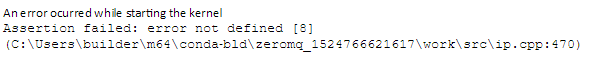Python 这个程序为什么运行过程中总是报错内存占用过大？ 40C
`````` import os
import numpy as np
mydi =os.listdir('G:\\百度导航轨迹数据\\8-9文件处理')
mydi = mydi[1:]

for ifile in mydi:
inputdir = open('G:\\百度导航数据  起始点经纬度存储\\8-9经纬度新.txt','a')
f1 = open('G:\\百度导航轨迹数据\\8-9文件处理'+'\\' + ifile)
alist = []
for line in f2:
line = line.strip()
line = line.split(',')
alist.append(line)
a = np.array(alist)
[rows,cols] = a.shape

odlist = []
pre = 0
inputdir.write(a + ',')
inputdir.write(a + ',')
if rows > 1:
for i in range(1,rows,15):
j = i
if int(a[i]) - int(a[pre]) > 515:
while i > pre:
#注意这一步可能有偏差，所以在下面要加上条件。
if int(a[i]) - int(a[i-1]) > 500:
break
else:
i -= 1
if int(a[i]) - int(a[i-1]) > 400:
inputdir.write(a[i]+',')
inputdir.write(a[i]+',')
pre = j
if int(a[rows - 1]) - int(a[i]) > 500:
inputdir.write(a[rows-1] + ',')
inputdir.write(a[rows - 1] + ',')
inputdir.write('\n')
else:
inputdir.write('\n')
inputdir.close()

``````2个回答

``````
import os
import numpy as np
mydi =os.listdir('G:\\百度导航轨迹数据\\8-9文件处理')
mydi = mydi[1:]

#改动一
'''创建一个生成器'''

for ifile in mydi:
inputdir = open('G:\\百度导航数据  起始点经纬度存储\\8-9经纬度新.txt','a')
f1 = open('G:\\百度导航轨迹数据\\8-9文件处理'+'\\' + ifile)

#改动二
#使用生成器

alist = []
for line in f2:
line = line.strip()
line = line.split(',')
alist.append(line)
a = np.array(alist)
[rows,cols] = a.shape

。。。省略。。。

``````